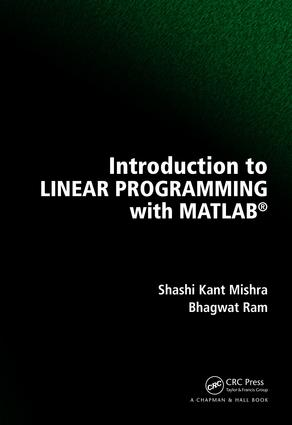# Introduction to Linear Programming with MATLAB

## 1st Edition

Chapman and Hall/CRC

313 pages

##### Purchasing Options:\$ = USD
Hardback: 9781138092266
pub: 2017-08-23
\$135.00
x
eBook (VitalSource) : 9781315104003
pub: 2017-09-07
from \$67.50

FREE Standard Shipping!

### Description

This book is based on the lecture notes of the author delivered to the students at the Institute of Science, Banaras Hindu University, India. It covers simplex, revised simplex, two-phase method, duality, dual simplex, complementary slackness, transportation and assignment problems with good number of examples, clear proofs, MATLAB codes and homework problems. The book will be useful for both students and practitioners.

### Table of Contents

Introduction. Introduction. Vector Spaces and Matrices. Vector. Matrix. Linear Equations. Matrix Inversion. Eigen values and Eigen vectors. Exercises. MATLAB. Introduction. Basic feature. Basic Operations in MATLAB. Selection Statements and Loop Statements. User Defined Function. MATLAB Functions Defined in This Book. Exercises. Introduction to Linear Programming. Introduction. Simple Examples of Linear programs. Convex Sets. Graphical Solution of Linear Programming problem. Exercises. Contents. The Simplex Method. Standard Form of Linear Programming Problem. Basic Solutions. Properties of Basic Solutions. Simplex Algorithm. Two Phase Simplex Method. Exercises. The Revised Simplex Method. Introduction. Matrix Form of the Revised Simplex Method. The Revised Simplex Algorithm. Exercise. Duality. Dual Linear Programs. Properties of Dual Problems. The Dual Simplex Method. Exercises. The Transportation Problem. Introduction. Balanced Transportation Problem. North West Corner Method. Least Cost Method. Vogel's Approximation Method. Optimal Solution from BFS. Exercises. The Assignment Problem. Introduction. Hungarian Method. Exercises. Answer Keys. Bibliography. Index

### About the Authors

Dr Shashi Kant Mishra is a Professor of Mathematics at the Institute of Science at Banaras Hindu Univeristy. His areas of research are generalized convexity, vector optimization, nonsmooth optimization and variational Inequalities.

### Subject Categories

##### BISAC Subject Codes/Headings:
BUS049000
BUSINESS & ECONOMICS / Operations Research
MAT003000
MATHEMATICS / Applied
TEC029000
TECHNOLOGY & ENGINEERING / Operations Research